Next: 3.5 Discussion Up: 3.4 Digit classification Previous: 3.4.1 Methods

## 3.4.2 Results

The results for the classification of the 28×28 digits are shown in table 3.1. The error rates for the two NGPCA variants were averaged over three separate training cycles (the difference between best and worst was around 0.2% for both variants). Both variants are better then a model using only a single PCA, and also better then Neural Gas with the same number of free parameters. MPPCA-ext could not be tested on this set because the large distances between digits lead to numerical zero probabilities (the maximum distance in a 784-dimensional cube of side length one is 28, this is large compared to a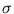of around 0.1).

Table 3.1: Classification performance on digits of size 28×28 from a training set composed of 60000 digits. The extension of Neural Gas to local PCA (NGPCA) is compared to PCA and Neural Gas.
 training method error NGPCA 2.79% NGPCA-constV 2.77% PCA 4.85% Neural Gas 4.22%

In the following, the ellipsoids of the NGPCA model are visualized (Möller and Hoffmann, 2004). Figure 3.10 shows the centers of the ten ellipsoids for each digit. Each center represents the local average over a subgroup of digits. Different ways to write a digit become visible, for example, digit 7' with or without a cross-bar.

The ellipsoid axis (eigenvectors) for one digit are visualized in figure 3.11. The eigenvectors represent variations around a center. This can be illustrated by adding multiples of an eigenvector to a center (figure 3.12). In the presented example, different sizes of the digit 2' are covered by the local PCA.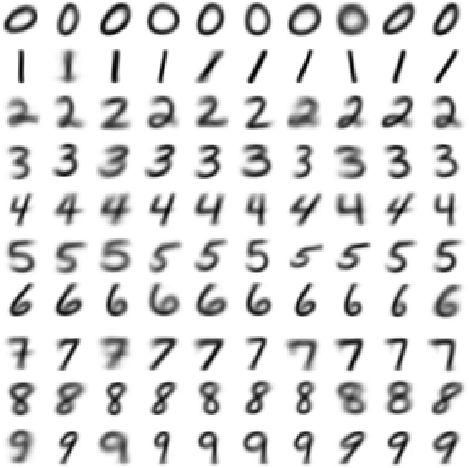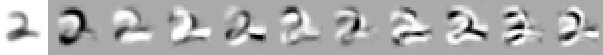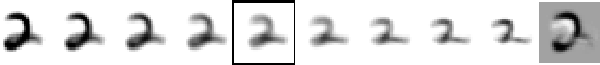Figure 3.13 shows a sample of mis-classified digits. Some of the mis-classified digits resemble the center they were assigned to (for example, the digit `9'). These digits seem to be extremes that lie close to representatives of another class.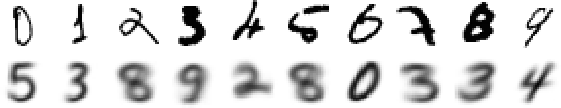The training set with digits of size 8×8 was used for a comparison with MPPCA-ext, and also for a comparison with local PCA mixture models from the literature (Hinton et al., 1997; Tipping and Bishop, 1999). These models worked on a different data set (CEDAR, which is commercial), however the size of the images ( 8×8) and the number of training patterns (1000 per digit) were the same. Moreover, these models had the same complexity as our models, namely ten units with ten principal components each. Tipping and Bishop (1999) used the discussed MPPCA model, and Hinton et al. (1997) used a mixture model that minimized the reconstruction error (as mentioned in section 2.3). Other mixture models that were tested on hand-written digits have a different complexity, for example, Meinicke and Ritter (2001) used a variable number of principal components. These models were excluded because they are hard to compare. Table 3.2 shows the result of the comparison. The errors from our models were averaged over three separate training cycles (the difference between worst and best was around 0.2%). Tipping and Bishop (1999) presented the result of the best training cycle.

Table 3.2: Classification performance on digits of size 8×8 from a training set composed of 10000 digits. Results are compared to two other local PCA mixture models that have the same complexity.
 training method database error NGPCA MNIST 4.78% NGPCA-constV MNIST 4.64% MPPCA-ext MNIST 4.58% Tipping and Bishop (1999) CEDAR 4.64% Hinton et al. (1997) CEDAR 4.91%Next: 3.5 Discussion Up: 3.4 Digit classification Previous: 3.4.1 Methods
Heiko Hoffmann
2005-03-22